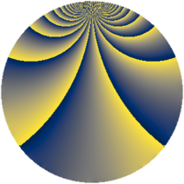# Properties

 Label 429.2.xLevel $429$ Weight $2$ Character orbit 429.x Rep. character $\chi_{429}(248,\cdot)$ Character field $\Q(\zeta_{10})$ Dimension $192$ Newform subspaces $1$ Sturm bound $112$ Trace bound $0$

# Related objects

## Defining parameters

 Level: $$N$$ $$=$$ $$429 = 3 \cdot 11 \cdot 13$$ Weight: $$k$$ $$=$$ $$2$$ Character orbit: $$[\chi]$$ $$=$$ 429.x (of order $$10$$ and degree $$4$$) Character conductor: $$\operatorname{cond}(\chi)$$ $$=$$ $$33$$ Character field: $$\Q(\zeta_{10})$$ Newform subspaces: $$1$$ Sturm bound: $$112$$ Trace bound: $$0$$

## Dimensions

The following table gives the dimensions of various subspaces of $$M_{2}(429, [\chi])$$.

Total New Old
Modular forms 240 192 48
Cusp forms 208 192 16
Eisenstein series 32 0 32

## Trace form

 $$192q + 6q^{3} - 48q^{4} - 10q^{6} - 14q^{9} + O(q^{10})$$ $$192q + 6q^{3} - 48q^{4} - 10q^{6} - 14q^{9} - 20q^{12} + 6q^{15} - 28q^{16} - 10q^{18} - 60q^{19} + 4q^{22} + 30q^{24} - 12q^{25} + 30q^{27} - 20q^{28} + 12q^{31} - 32q^{33} + 56q^{34} + 44q^{36} + 60q^{40} - 108q^{42} - 40q^{45} - 28q^{48} - 24q^{49} + 10q^{51} + 20q^{52} - 20q^{55} + 50q^{57} - 60q^{58} + 36q^{60} + 20q^{61} - 30q^{63} + 64q^{64} - 30q^{66} - 120q^{67} - 16q^{69} + 20q^{70} + 150q^{72} - 20q^{73} + 18q^{75} + 40q^{78} - 160q^{79} - 46q^{81} + 88q^{82} - 40q^{85} + 72q^{88} - 80q^{90} + 8q^{91} - 72q^{93} - 120q^{94} - 140q^{96} - 88q^{97} + 62q^{99} + O(q^{100})$$

## Decomposition of $$S_{2}^{\mathrm{new}}(429, [\chi])$$ into newform subspaces

Label Dim. $$A$$ Field CM Traces $q$-expansion
$$a_2$$ $$a_3$$ $$a_5$$ $$a_7$$
429.2.x.a $$192$$ $$3.426$$ None $$0$$ $$6$$ $$0$$ $$0$$

## Decomposition of $$S_{2}^{\mathrm{old}}(429, [\chi])$$ into lower level spaces

$$S_{2}^{\mathrm{old}}(429, [\chi]) \cong$$ $$S_{2}^{\mathrm{new}}(33, [\chi])$$$$^{\oplus 2}$$